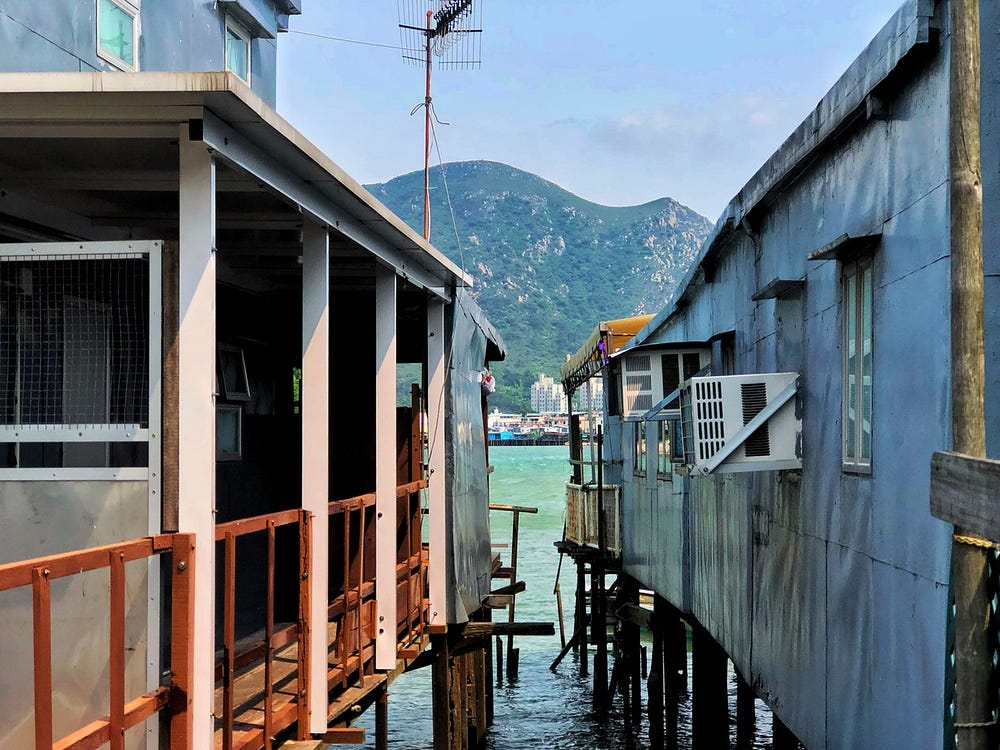# How Fast R-CNN works on object detection?

Source: Deep Learning on MediumThis is the second story for R-CNN series. You may understand more about R-CN from here. Fast R-CNN (Region-based Convolutional Neural Network) is designed to tackle the object detection problems.

This story will discuss Fast R-CNN (Girshick, 2015), and the following will be covered:

• The architecture of Fast R-CNN
• Region-of-Interest Pooling (RoIPool)
• Model Training
• Experiment

# Architecture

Giving an image and region proposals, it will passing thought convolutional network, Region-of-Interest (RoI) polling, fully connected network networks (FC) and the final output are the probability of object class and corresponding bounding box positions.

To prevent missing lots of objects, it is intended to have a high recall in finding region proposals. However, it impacts the performance in object detection parts. RoI comes to address this issue by choosing suitable region proposals.

# Region-of-Interest Pooling (RoIPool)

RoI pooling is the trick to improve the issue in R-CNN. Instead of the re-calculating a similar region again and again. RoI aims to reduce the computational complexity to speed up the process.

It uses max-pooling to extract interested feature maps from a big feature map. This interesting feature map is fixed per pooling layer. In Fast R-CNN, the input of RoI pooling comes from selective search while the output is a list of image index and bounding box (top left and bottom right). So we have Nx5 (N: number of RoI) outputs

For every RoI, it scales the input to pre-defined (e.g. 2×2) size. The procedures are:

• `input`: Having a feature map
• `polling sections`: Dividing the region proposals to a dimension of the output (e.g. 2×2 for this example)
• `max values in sections`: Applying the max-pooling concept to retrieve the highest value
• `output`: a small size feature map

Here is a detail explanation of RoI polling.

# Model Training

## Loss Function

A Fast R-CNN includes two outputs which are object class probability (classification) and bounding box offsets (regression). There are not trained separately but training both classifier and regressor together.

• L`cls`: Classifier loss
• L`loc`: Regressor loss
• u: ground-truth class
• v: ground-truth bounding box

## Mini-batch Sampling

Sampling 64 RoIs from each image and assigned label to those region proposals according to the following criteria.

Positive Label:

• If the overlapping rate (between region proposals and ground-truth box) is higher than 0.5, it will treat as valid region proposals.
• Selecting 25% of valid region proposals.

Negative Label:

• If the overlapping rate is between 0.1 and 0.5, selecting the maximum one.

# Experiments

Fast R-CNN gets better in most of the object class.

# Take Away

• Multi-task training (object classification and bounding box regression) prevents multi-stage training and prediction.
• More proposals are better because the model cannot classify the object if there are no region proposals.